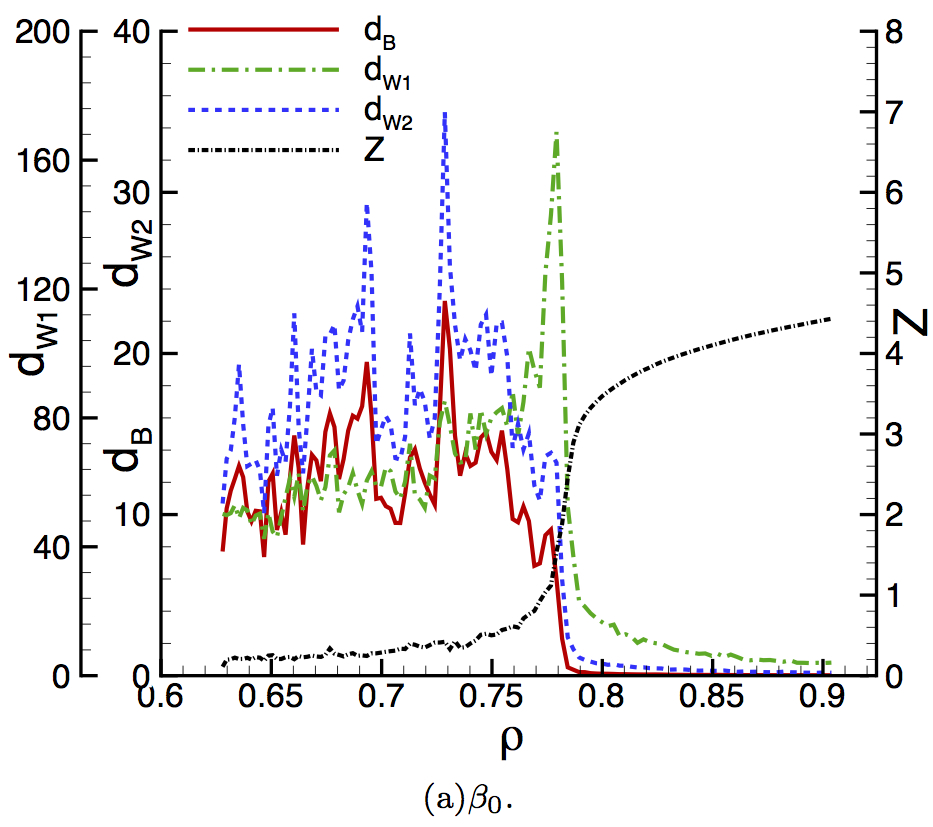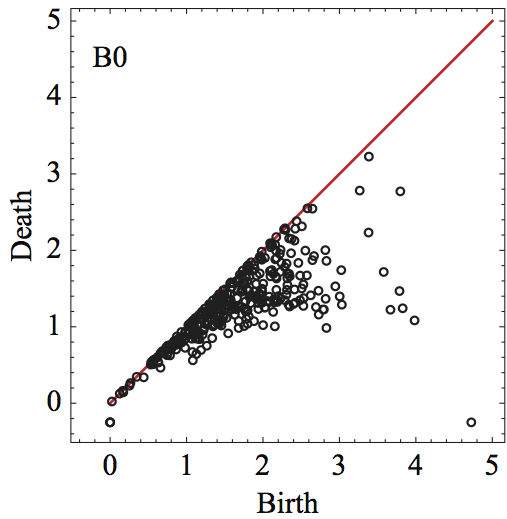Topological Characterization of Dense Granular Media

Overview

Particulate systems consisting of a large number of particles have attracted significant attention in the last decades. Despite significant research on these systems,  their properties are still not well understood and some of them appear to be rather elusive.  The fact that the forces do not propagate uniformly in the systems made of interacting particles has been established in a number of different systems, ranging from those where particles' are on atomic  or molecular scales, to those with macroscopic particles. Very recently, initial attempts have been made to consider the inter particle forces based on network-type of analysis, and of by exploring their topological properties.
With the goal of extending our ability to systematically explore network properties in greater detail, we developed rigorous mathematical models capable of capturing geometric features of  particle interactions. The approach  is based on algebraic topology and in particular persistent homology.  This is  a relatively new mathematical technique that provides a computationally efficient rigorous framework for  multi scale analysis.

Publications

Evolution of Force Networks in Dense Particulate MediaAbstract

We introduce novel sets of measures with the goal of describing dynamical properties of force networks in dense particulate systems. The presented approach is based on persistent homology and allows for extracting precise, quantitative measures that describe the evolution of geometric features of the interparticle forces, without necessarily considering the details related to individual contacts between particles. The networks considered emerge from discrete element simulations of two dimensional particulate systems consisting of compressible frictional circular disks. We quantify the evolution of the networks for slowly compressed systems undergoing jamming transition. The main findings include uncovering significant but localized changes of force networks for unjammed systems, global (system-wide) changes as the systems evolve through jamming, to be followed by significantly less dramatic evolution for the jammed states. We consider both connected components, related in loose sense to force chains, and loops, and find that both measures provide a significant insight into the evolution of force networks. In addition to normal, we consider also tangential forces between the particles and find that they evolve in the consistent manner. Consideration of both frictional and frictionless systems leads us to the conclusion that friction plays a significant role in determining the dynamical properties of the considered networks. We find that the proposed approach describes the considered networks in a precise yet tractable manner, allowing to identify novel features which could be difficult or impossible to describe using other approaches.

Papers

Quantifying force networks in particulate systemsAbstract

We present mathematical models based on persistent homology for analyzing force distributions in particulate sys- tems. We define three distinct chain complexes of these distributions: digital, position, and interaction, motivated by different types of data that may be available from experiments and simulations, e.g. digital images, location of the particles, and the forces between particles, respectively. We describe how algebraic topology, in particular, homology allows one to obtain algebraic representations of the geometry captured by these complexes. For each complex we define an associated force network from which persistent homology is computed. Using numerical data obtained from discrete element simulations of a system of particles undergoing slow compression, we demonstrate how persistent homology can be used to compare the force distributions in different systems, and discuss the differences between the properties of digital, position, and interaction force networks. To conclude, we formulate well-defined measures quantifying differences between force networks corresponding to different states of a system, and therefore allow to analyze in precise terms dynamical properties of force networks.

Paper

Persistence of force networks in compressed granular mediaAbstract

We utilize the tools of persistent homology to analyze features of force networks in dense granular matter, modeled as a collection of circular, inelastic frictional particles. The proposed approach describes these networks in a precise and tractable manner, allowing us to identify features that are difficult or impossible to characterize by other means. In contrast to other techniques that consider each force threshold level separately, persistent homology allows us to consider all threshold levels at once to describe the force network in a complete and insightful manner. We consider continuously compressed system of particles characterized by varied polydispersity and friction in two spatial dimensions. We find significant differences between the force networks in these systems, suggesting that their mechanical response may differ considerably as well.